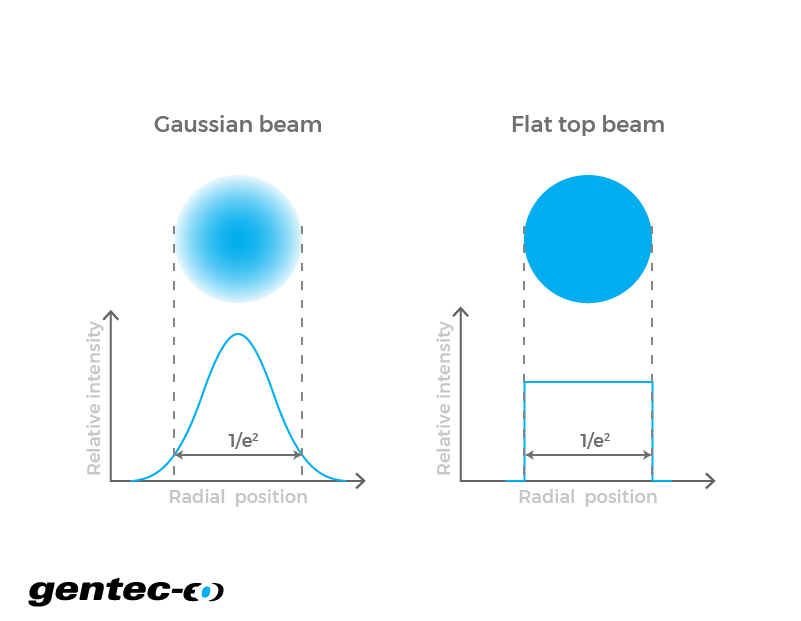EN

# Energy density / Fluence

Input basic beam parameters about your laser and let our tool calculates its energy density in a second.

- cm2

- J/cm2

- J

## How does the laser energy density calculator work?

In a pulsed laser, the beam is separated in multiple peaks of emission. All these pulses have discrete values of energy. Similar to the calculation of the power density, the average energy density corresponds to the total energy in each pulse divided by the beam size on a given surface. Conversely, if you know the average power of your laser and the rate at which it emits pulses, you can determine the energy in each pulse. Therefore, you can calculate its energy density or fluence. This value is important to consider since, despite having a low average power, a laser could have too much energy in each pulse for a given target to withstand it. So each pulse could damage the material.

Click to
enlarge## Energy density formulas

The formulas describe the behavior of a theoretical flat-top or a perfectly Gaussian laser beam. In such, they represent an approximation of the values one would obtain in real conditions. Also, there are multiple methods one can use to measure the diameter of a Gaussian beam. The reason for this mainly comes from the fact that its theoretical value only reaches 0 when the radius reaches infinity. Therefore, the beam would have an infinite diameter. So, we chose to use the method where it is measured using the 1/e² parameter. At this point, the beam diameter is approximately 1.699 times the full diameter measured at half the maximum of a Gaussian function (FWHM). At 1/e², it represents approximately 86.5% of the total power. Note that for a flat-top beam, the formulas are used just as is, but for a Gaussian beam, there is a factor 2 that multiplies the right hand part of these equations.

$$\text{Energy density} \left(\frac{J}{cm^2}\right) = \frac{\text{Average power}(W)}{\text{Repetition rate}(Hz) \times \text{Beam area}(cm^2)}$$

Right-click
to copy

$$\text{Energy density} \left(\frac{J}{cm^2}\right) = \frac{\text{Energy per pulse}(J)}{\text{Beam area}(cm^2)}$$

Right-click
to copy